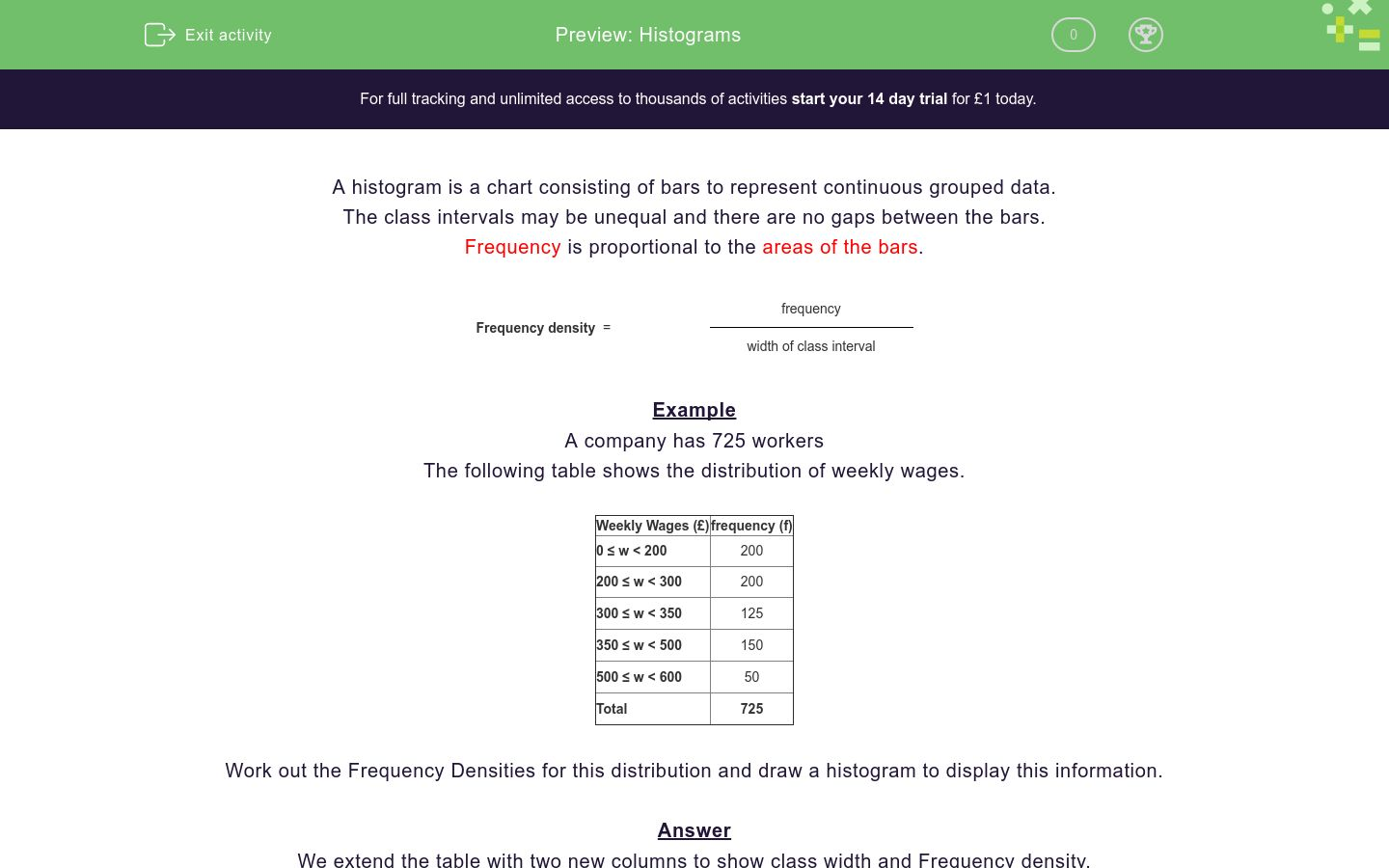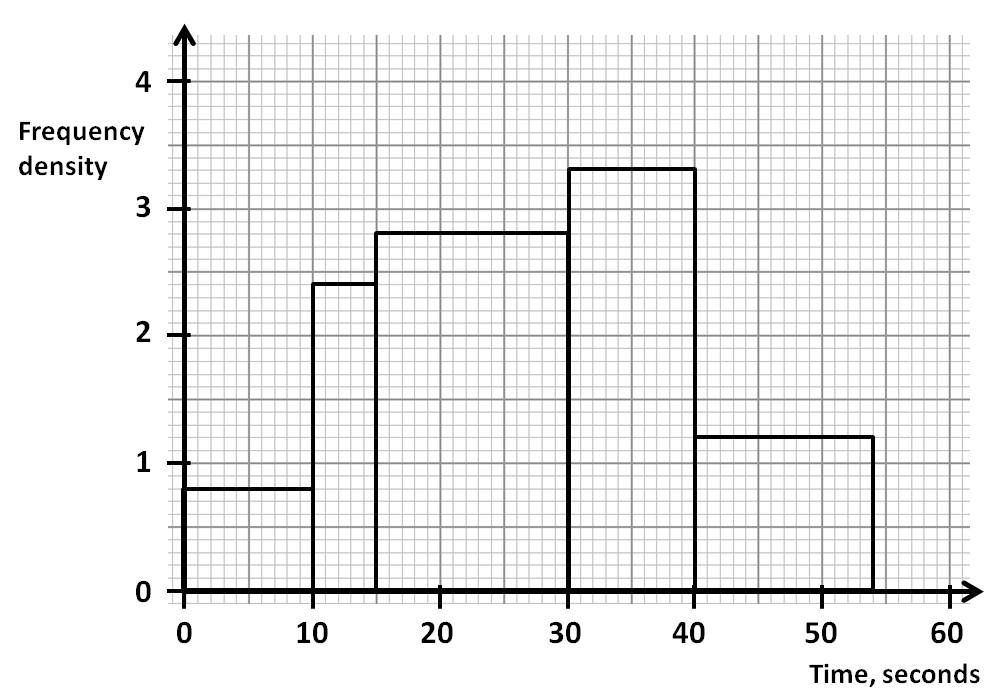# Histograms

In this worksheet, students understand frequency densities and how to read information from a histogram and its associated table of data.Key stage:  KS 4

Curriculum topic:  Statistics

Curriculum subtopic:  Interpret and Construct Tables and Line Graphs for Time Series Data

Difficulty level:### QUESTION 1 of 10

A histogram is a chart consisting of bars to represent continuous grouped data.

The class intervals may be unequal and there are no gaps between the bars.

Frequency is proportional to the areas of the bars.

 Frequency density  = frequency width of class interval

Example

A company has 725 workers

The following table shows the distribution of weekly wages.

Weekly Wages (£) frequency (f)
0 ≤ w < 200 200
200 ≤ w < 300 200
300 ≤ w < 350 125
350 ≤ w < 500 150
500 ≤ w < 600 50
Total 725

Work out the Frequency Densities for this distribution and draw a histogram to display this information.

We extend the table with two new columns to show class width and Frequency density.

Using the formula below, we work out the Frequency density for each class width.

 Frequency density  = frequency width of class interval

Weekly Wages (£) frequency (f) Class width Frequency density
0 ≤ w < 200 200 200   200 ÷ 200 = 1
200 ≤ w < 300 200 100   200 ÷ 100 = 2
300 ≤ w < 350 125 50   125 ÷ 50 = 2.5
350 ≤ w < 500 150 150   150 ÷ 150 = 1
500 ≤ w < 600 50 100   50 ÷ 100 = 0.5
Total  725

Weekly wages are then plotted against Frequency density to produce the following histogram.For this worksheet, you will need the formula:

Frequency = Frequency Density × Class Width

A company has 725 workers.

The following histogram shows the distribution of weekly wages.

Work out the frequency for the 0 ≤ w < 200 class interval.A company has 725 workers.

The following histogram shows the distribution of weekly wages.

Work out the frequency for the 300 ≤ w < 350 class interval.A company has 725 workers.

The following histogram shows the distribution of weekly wages.

Work out the frequency for the 350 ≤ w < 500 class interval.The following histogram shows the distribution of ages of people staying at a hotel.

Work out the frequency for the 10 ≤ a < 25 class interval.The following histogram shows the distribution of ages of people staying at a hotel.

Work out the frequency for the 30 ≤ a < 40 class interval.The following histogram shows the distribution of ages of people staying at a hotel.

Work out the frequency for the 25 ≤ a < 30 class interval.The following histogram shows the distribution of ages of people staying at a hotel.

Work out the frequency for the 40 ≤ a < 55 class interval.The following histogram shows the distribution of the lengths of adverts on one television channel during a particular day.

Work out the frequency for the 0 ≤ t < 10 class interval.The following histogram shows the distribution of the lengths of adverts on one television channel during a particular day.

Work out the frequency for the 10 ≤ t < 15 class interval.The following histogram shows the distribution of the lengths of adverts on one television channel during a particular day.

Work out how many TV adverts have been surveyed in total.• Question 1

A company has 725 workers.

The following histogram shows the distribution of weekly wages.

Work out the frequency for the 0 ≤ w < 200 class interval.200
EDDIE SAYS
Class width = 200 - 0 = 200
Frequency = Frequency Density × Class Width = 1 × 200 = 200
• Question 2

A company has 725 workers.

The following histogram shows the distribution of weekly wages.

Work out the frequency for the 300 ≤ w < 350 class interval.125
EDDIE SAYS
Class width = 350 - 300 = 50
Frequency = Frequency Density × Class Width = 2.5 × 50 = 125
• Question 3

A company has 725 workers.

The following histogram shows the distribution of weekly wages.

Work out the frequency for the 350 ≤ w < 500 class interval.150
EDDIE SAYS
Class width = 500 - 350 = 150
Frequency = Frequency Density × Class Width = 1 × 150 = 150
• Question 4

The following histogram shows the distribution of ages of people staying at a hotel.

Work out the frequency for the 10 ≤ a < 25 class interval.30
EDDIE SAYS
Class width = 25 - 10 = 15
Frequency = Frequency Density × Class Width = 2 × 15 = 30
• Question 5

The following histogram shows the distribution of ages of people staying at a hotel.

Work out the frequency for the 30 ≤ a < 40 class interval.35
EDDIE SAYS
Class width = 40 - 30 = 10
Frequency = Frequency Density × Class Width = 3.5 × 10 = 35
• Question 6

The following histogram shows the distribution of ages of people staying at a hotel.

Work out the frequency for the 25 ≤ a < 30 class interval.13
EDDIE SAYS
Class width = 30 - 25 = 5
Frequency = Frequency Density × Class Width = 2.6 × 5 = 13
• Question 7

The following histogram shows the distribution of ages of people staying at a hotel.

Work out the frequency for the 40 ≤ a < 55 class interval.18
EDDIE SAYS
Class width = 55 - 40 = 15
Frequency = Frequency Density × Class Width = 1.2 × 15 = 18
• Question 8

The following histogram shows the distribution of the lengths of adverts on one television channel during a particular day.

Work out the frequency for the 0 ≤ t < 10 class interval.8
EDDIE SAYS
Class width = 10 - 0 = 10
Frequency = Frequency Density × Class Width = 0.8 × 10 = 8
• Question 9

The following histogram shows the distribution of the lengths of adverts on one television channel during a particular day.

Work out the frequency for the 10 ≤ t < 15 class interval.12
EDDIE SAYS
Class width = 15 - 10 = 5
Frequency = Frequency Density × Class Width = 2.4 × 5 = 12
• Question 10

The following histogram shows the distribution of the lengths of adverts on one television channel during a particular day.

Work out how many TV adverts have been surveyed in total.113
EDDIE SAYS
We add up all the frequencies to get:
(0.8 × 10) + (2.4 × 5) + (2.8 × 15) + (3.3 × 10) + (1.2 × 15)
= 8 + 12 + 42 + 33 + 18 = 113
---- OR ----

Sign up for a £1 trial so you can track and measure your child's progress on this activity.

### What is EdPlace?

We're your National Curriculum aligned online education content provider helping each child succeed in English, maths and science from year 1 to GCSE. With an EdPlace account you’ll be able to track and measure progress, helping each child achieve their best. We build confidence and attainment by personalising each child’s learning at a level that suits them.

Start your £1 trial